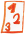Math worksheetsCustom math worksheets at your fingertips

# Details for problem "Examine the elements of binomic formulas"

Quickname: 7596

## Summary

Elements of a binomic formula and their expanded form are grouped in a table. Fill the empty spaces.

## Example## Description

In a tabular form, structural elements of binomic formulas in parentheses and expanded form like

- binomic formular in parentheses form
- a
- b
- 2ab
- a^2
- b^2
- expanded form

are shown as captions for a table. The task is, for a given binomic formular, to identify these structural elements and fill in the table's columns accordingly.

In every row of the table, depending on the problem statement, some elements will be given, some will be left blank to be filled in.

Fot the problem generation, it is selectable if a and b will be simple literal numbers or variables or multiples of variables. The first problem may serve as an example and will then presented as a fully populated row.

The number of problems is selectable, and the types of formulas to be used.

Download free worksheets for this math problem here. There is a second sheet with the solutions. Just click on the respective link.Worksheet 1Solution sheetWorksheet 2Solution sheetWorksheet 3Solution sheet

If you can not see the solution sheets for download, they may be filtered out by an ad blocker that you may have installed. If this is the case, please allow ads for this page and reload the page. The solution sheets will then reappear.

• Do the sample worksheets do not really fit?
• Do you need more math worksheets, with a different level of difficulty?
• Would you like to combine different problems on a worksheet and adjust them to your needs?
• As a teacher, you can put together your own worksheets using the automatically generated math problems provided.
With a free initial credit, you can start creating your own math worksheets in a few minutes.

It does not cost anything to try! Register here, to create custom worksheets now!

## Customization options for this problem

ParameterPossible values
Number of problems1, 2, 3, 4, 5, 6, 7, 8, 9, 10
Type of formula(a+b)^2 & (a-b)^2, (a+b)(a-b)
Columns providedparentheses form, expanded, a,b, a^2, b^2, mix of a,a^2,b,b^2, random
Summandssimple var/number, product of var/number
Sample problemYes, No